# Goniometry and trigonometry - math word problems

#### Number of problems found: 336

• ForcesIn point O acts three orthogonal forces: F1 = 20 N, F2 = 7 N, and F3 = 19 N. Determine the resultant of F and the angles between F and forces F1, F2, and F3.
• DiagonalDetermine the dimensions of the cuboid, if diagonal long 53 dm has an angle with one edge 42° and with another edge 64°.
• RightDetermine angles of the right triangle with the hypotenuse c and legs a, b, if: 3a +4b = 4.9c
• ClimbRoad has climbing 1:27. How big is a angle corresponds to this climbing?
• PentagonCalculate the area of a regular pentagon, which diagonal is u=16.
• Statue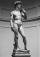On the pedestal high 4 m is a statue 2.7 m high. At what distance from the statue must the observer stand to see it at the maximum viewing angle? Distance from the eye of the observer from the ground is 1.7 m.
• CuboidFind the dimensions of cuboid a, b, c; if diagonal d=9 dm has an angle with edge α=55° and has an angle with edge b β=58°
• Triangle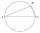Calculate the area of the right triangle ΔABC if one leg is long 14 and its opposite angle is 59°.
• Angle between lines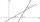Calculate the angle between these two lines: p: -8x +4y +5 =0 q: 10x +10y -7=0
• MastMast has 13 m long shadow on a slope rising from the mast foot in the direction of the shadow angle at angle 13.3°. Determine the height of the mast, if the sun above the horizon is at angle 45°12'.
• Felix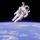Calculate how much land saw Felix Baumgartner after jump from 32 km above ground. The radius of the Earth is R = 6378 km.
• Arc and segmentCalculate the length of circular arc l, area of the circular arc S1 and area of circular segment S2. Radius of the circle is 11 and corresponding angle is (2)/(12) π.
• Height 2Calculate the height of the equilateral triangle with side 27.
• Trigonometric functionsIn the right triangle is: tg α= frac(2) 1 Find the value of s and c: sin α= (s)/(√ 5) cos α= (c)/(√ 5)
• TrigonometryIs true equality? sin(x +13 π)= sin(x)
• BoxCalculate the angle between box base 9 x 14 and body diagonal length 18.
• The angle of lines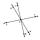Calculate the angle of two lines y=x-21 and y=-2x+14
• V-belt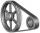Calculate the length of the belt on pulleys with diameters of 105 mm and 393 mm at shaft distance 697 mm.
• Earth parallelEarth's radius is 6370 km long. Calculate the length parallel of latitude 50°.
• TrigonometryIf you know that cos(γ) = sin (806°), what is the angle γ?

Do you have an exciting math question or word problem that you can't solve? Ask a question or post a math problem, and we can try to solve it.

We will send a solution to your e-mail address. Solved examples are also published here. Please enter the e-mail correctly and check whether you don't have a full mailbox.

Please do not submit problems from current active competitions such as Mathematical Olympiad, correspondence seminars etc...

Most natural application of trigonometry and trigonometric functions is a calculation of the triangles. Common and less common calculations of different types of triangles offers our triangle calculator. Word trigonometry comes from Greek and literally means triangle calculation.